# Electronics and Communication Engineering - Exam Questions Papers

1.
The electric field of 2.5 x 107 V/m can give 1 eV energy to condition electron, then in a copper block what will be the mean free path of electron?
5 x 10-8 m
4 x 10-7 m
0.4 m
0.5 m
Explanation:

The force on electron in eE. As the electron moves through distance d , the work done on it is eEd.

This is equal to energy transferred to electron.

eEd = 1 eV

d = 4 x 10-7m.

2.
25 persons are in a room. 15 of them play hockey, 17 of them play football and 10 of them play both hockey and football. Then the number of persons playing neither hockey nor football is:
2
17
13
3
Explanation:

Representing the given information in the Venn diagrams, we have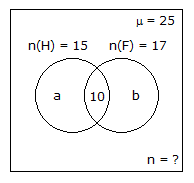Let the number of people who play only hockey = a

The number of people who play only football = b

Now, a = n(H) - 10 = 15 - 10 = 5

b = n(F) - 10 = 17 - 10 = 7

Clearly, a + b + 10 + n = 25

n = 25 - 7 - 5 -10 ⇒ n = 3

The number of people who do not play neither Hockey nor Football is 3.

3.
For the circuit shown in the following figure I0-I3 are inputs to the 4:1 multiplexer R(MSB) and S are control bits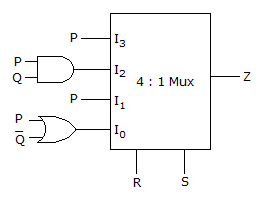The output Z can be represented by
PQ + PQ S + Q R S
P Q + PQ R + P Q S
P Q R + P QR + PQRS + Q R S
PQ R + PQR S + P Q R S + Q R S
Explanation:

Use this to find solution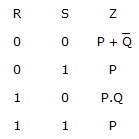4.
The transfer function of a system is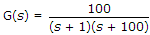. For a unit-step input to the system the approximate setting time for 2% criterion is :
100 sec
4 sec
1 sec
0.01 sec
Explanation: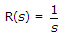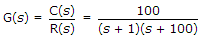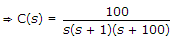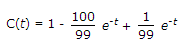ξωn = 1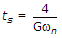for 2% = 4 sec.

5.
The Nyquist sampling interval, for the signal sine (700t) + sine (500t) is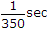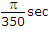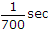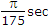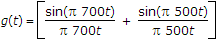Then Nyquist interval is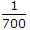sec.